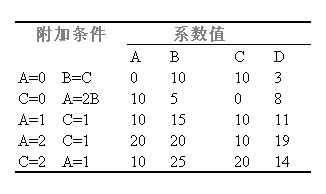# 化学自习室移动版

### 关于有多套配平系的氧化还原方程式

13H2SO4+10KSCN+12KMnO4=12MnSO4+11K2SO4+10HCN+8H2O

2KClO3+4HCl=2KCl+2H2O+Cl2+2ClO2  (1)

11KClO3+18HCl=11KCl+9H2O+3Cl2+12ClO2  (2)

8KClO3+24HCl=8KCl+12H2O+9Cl2+6ClO2  (3)

上面提到的KClO3HCl的反应，实际上是由二个配平了的独立反应式组成：

KClO3+6HCl=KCl+3H2O+3Cl2                           (4)

5KClO3+6HCl=5KCl+3H2O+6ClO2                     (5)

(x+5y)KClO3+6(x+y)HCl=(x+5y)KCl+3(x+y)H2O+3xCl2+6yClO2  (6)

在酸性介质中H2O2KMnO4的反应。

5H2O2+2KMnO4+3H2SO4=2Mn2SO4+5O2+K2SO4+8H2O  (7)

7H2O2+2KMnO4+3H2SO4=2MnSO4+6O2+K2SO4+10H2O  (8)

12H2O2+4KMnO4+6H2SO4=4MnSO4+11O2+2K2SO4+18H2O  (9)

……等。

2H2O2=2H2O+O2  (10)

(5x+2y)H2O2+2xKMnO4+3xH2SO4=xMnSO4+(5x+y)O2

+xK2SO4+(8x+2y)H2O  (11)

〔类例1

3HClO3=HClO4+Cl2+2O2+H2O  (12)

7HClO3=5HClO4+Cl2+H2O  (13)

4HClO3=2Cl2+5O2+2H2O  (14)

〔类例2

3SO2+7C=CS2+S+6CO  (16)

SO2+2C=S+2CO  (17)

2SO2+5C=CS2+4CO  (18)

〔类例3

NaClO+H2O2=NaCl+H2O+O2  (19)

二、氧化数变化值易混淆的氧化还原方程式

P2I4+P4+H2OPH4I+H3PO4   (未配平)                    (20)

()Carrano方法〔3Carrano建议使用代数方法求解，其具体步骤如下：

1步〕假设反应涉及到四个半反应

[P2I4+8H++10e-=2PH4I+2I-]×A                                    (21)

[P4+4I-+16H++12e-=4PH4I]×B                                     (22)

[P2I4+8H2O=2H3PO4+4I-+10H++6e-]×C                         (23)

[P4+16H2O=4H3PO4+20H++20e-]×D                             (24)

2步〕基于方程二边的电子、质子和碘离子的相等可建立三个联立方程组：

10A12B=6C20D                                                 (25)

8A16B10C20D                                               (26)

4B2A4C                                                            (27)

ABCD分别表示四个半反应系数前的乘数。

3步〕确定ABCD以求出配平系数。

10P2I4+13P4+128H2O=40PH4I+32H3PO4  (28)

1系数值的说明例附加条件()Mayper方法

Mayper认为Carrano方法（半反应+代数法）过于麻烦，可直接使用简单的代数方法，即：

aP2I4+bP4+cH2O=dPH4I+eH3PO4  (29)

P2a+4b=d+3

I4a=d

H2c=4d+3e

Oc=4e

(责任编辑：)• 全部评论（0
还没有评论，快来抢沙发吧！
相关文章
作者其他文章
最近关注
热点内容
公益广告